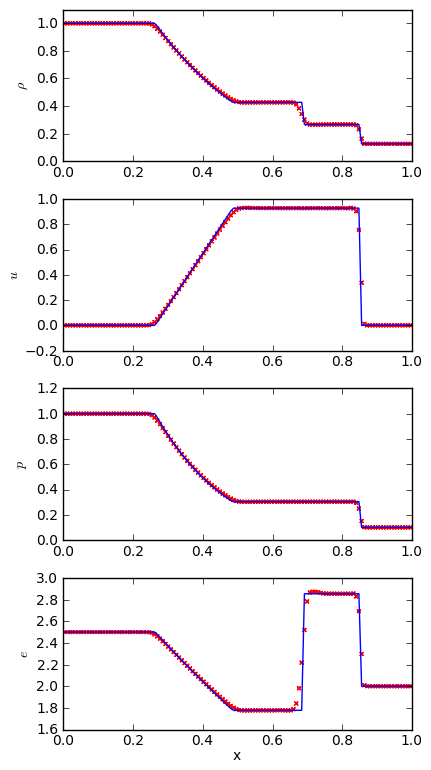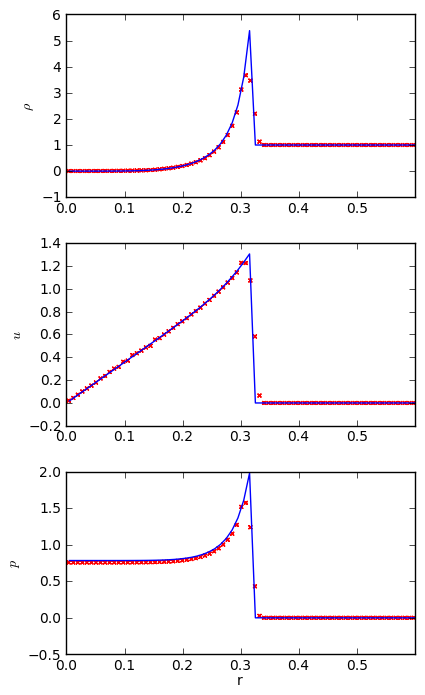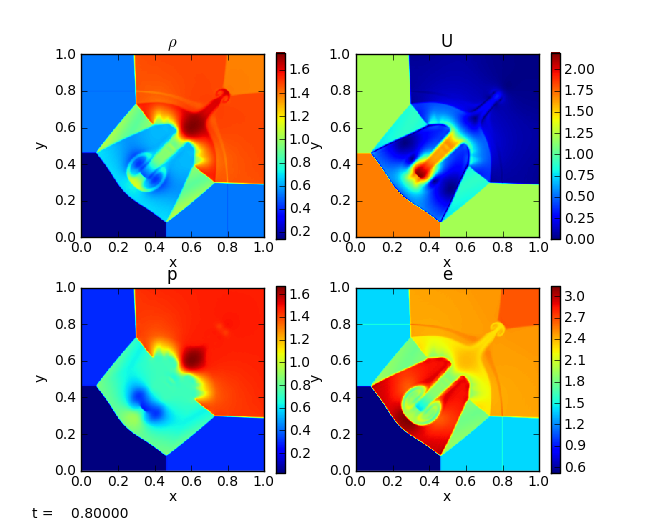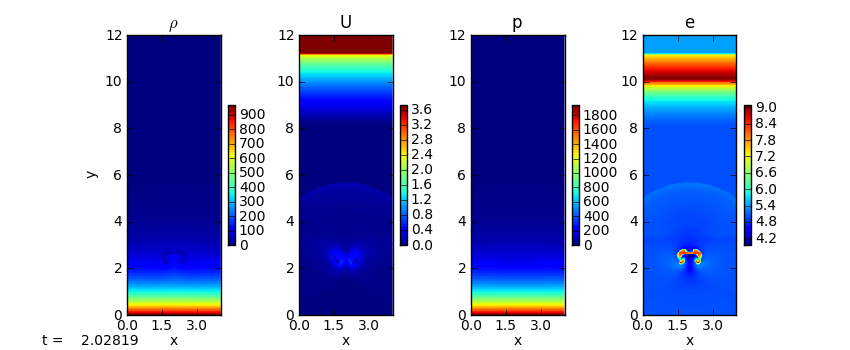# Compressible hydrodynamics solvers¶

The Euler equations of compressible hydrodynamics take the form:

$\begin{split}\frac{\partial \rho}{\partial t} + \nabla \cdot (\rho U) &= 0 \\ \frac{\partial (\rho U)}{\partial t} + \nabla \cdot (\rho U U) + \nabla p &= \rho g \\ \frac{\partial (\rho E)}{\partial t} + \nabla \cdot [(\rho E + p ) U] &= \rho U \cdot g\end{split}$

with $$\rho E = \rho e + \frac{1}{2} \rho |U|^2$$ and $$p = p(\rho, e)$$. Note these do not include any dissipation terms, since they are usually negligible in astrophysics.

pyro has several compressible solvers to solve this equation set. The implementations here have flattening at shocks, artificial viscosity, a simple gamma-law equation of state, and (in some cases) a choice of Riemann solvers. Optional constant gravity in the vertical direction is allowed.

Note

All the compressible solvers share the same problems/ directory, which lives in compressible/problems/. For the other compressible solvers, we simply use a symbolic-link to this directory in the solver’s directory.

## compressible solver¶

compressible is based on a directionally unsplit (the corner transport upwind algorithm) piecewise linear method for the Euler equations, following . This is overall second-order accurate.

The parameters for this solver are:

• section: [compressible]

option

value

description

use_flattening

1

apply flattening at shocks (1)

z0

0.75

flattening z0 parameter

z1

0.85

flattening z1 parameter

delta

0.33

flattening delta parameter

cvisc

0.1

artifical viscosity coefficient

limiter

2

limiter (0 = none, 1 = 2nd order, 2 = 4th order)

grav

0.0

gravitational acceleration (in y-direction)

riemann

HLLC

HLLC or CGF

• section: [driver]

option

value

description

cfl

0.8

• section: [eos]

option

value

description

gamma

1.4

pres = rho ener (gamma - 1)

• section: [particles]

option

value

description

do_particles

0

particle_generator

grid

## compressible_rk solver¶

compressible_rk uses a method of lines time-integration approach with piecewise linear spatial reconstruction for the Euler equations. This is overall second-order accurate.

The parameters for this solver are:

• section: [compressible]

option

value

description

use_flattening

1

apply flattening at shocks (1)

z0

0.75

flattening z0 parameter

z1

0.85

flattening z1 parameter

delta

0.33

flattening delta parameter

cvisc

0.1

artifical viscosity coefficient

limiter

2

limiter (0 = none, 1 = 2nd order, 2 = 4th order)

temporal_method

RK4

integration method (see mesh/integration.py)

grav

0.0

gravitational acceleration (in y-direction)

riemann

HLLC

HLLC or CGF

well_balanced

0

use a well-balanced scheme to keep the model in hydrostatic equilibrium

• section: [driver]

option

value

description

cfl

0.8

• section: [eos]

option

value

description

gamma

1.4

pres = rho ener (gamma - 1)

## compressible_fv4 solver¶

compressible_fv4 uses a 4th order accurate method with RK4 time integration, following .

The parameter for this solver are:

• section: [compressible]

option

value

description

use_flattening

1

apply flattening at shocks (1)

z0

0.75

flattening z0 parameter

z1

0.85

flattening z1 parameter

delta

0.33

flattening delta parameter

cvisc

0.1

artifical viscosity coefficient

limiter

2

limiter (0 = none, 1 = 2nd order, 2 = 4th order)

temporal_method

RK4

integration method (see mesh/integration.py)

grav

0.0

gravitational acceleration (in y-direction)

• section: [driver]

option

value

description

cfl

0.8

• section: [eos]

option

value

description

gamma

1.4

pres = rho ener (gamma - 1)

## compressible_sdc solver¶

compressible_sdc uses a 4th order accurate method with spectral-deferred correction (SDC) for the time integration. This shares much in common with the compressible_fv4 solver, aside from how the time-integration is handled.

The parameters for this solver are:

• section: [compressible]

option

value

description

use_flattening

1

apply flattening at shocks (1)

z0

0.75

flattening z0 parameter

z1

0.85

flattening z1 parameter

delta

0.33

flattening delta parameter

cvisc

0.1

artifical viscosity coefficient

limiter

2

limiter (0 = none, 1 = 2nd order, 2 = 4th order)

temporal_method

RK4

integration method (see mesh/integration.py)

grav

0.0

gravitational acceleration (in y-direction)

• section: [driver]

option

value

description

cfl

0.8

• section: [eos]

option

value

description

gamma

1.4

pres = rho ener (gamma - 1)

## Example problems¶

Note

The 4th-order accurate solver (compressible_fv4) requires that the initialization create cell-averages accurate to 4th-order. To allow for all the solvers to use the same problem setups, we assume that the initialization routines initialize cell-centers (which is fine for 2nd-order accuracy), and the preevolve() method will convert these to cell-averages automatically after initialization.

### Sod¶

The Sod problem is a standard hydrodynamics problem. It is a one-dimensional shock tube (two states separated by an interface), that exhibits all three hydrodynamic waves: a shock, contact, and rarefaction. Furthermore, there are exact solutions for a gamma-law equation of state, so we can check our solution against these exact solutions. See Toro’s book for details on this problem and the exact Riemann solver.

Because it is one-dimensional, we run it in narrow domains in the x- or y-directions. It can be run as:

./pyro.py compressible sod inputs.sod.x
./pyro.py compressible sod inputs.sod.y


A simple script, sod_compare.py in analysis/ will read a pyro output file and plot the solution over the exact Sod solution. Below we see the result for a Sod run with 128 points in the x-direction, gamma = 1.4, and run until t = 0.2 s.We see excellent agreement for all quantities. The shock wave is very steep, as expected. The contact wave is smeared out over ~5 zones—this is discussed in the notes above, and can be improved in the PPM method with contact steepening.

### Sedov¶

The Sedov blast wave problem is another standard test with an analytic solution (Sedov 1959). A lot of energy is point into a point in a uniform medium and a blast wave propagates outward. The Sedov problem is run as:

./pyro.py compressible sedov inputs.sedov


The video below shows the output from a 128 x 128 grid with the energy put in a radius of 0.0125 surrounding the center of the domain. A gamma-law EOS with gamma = 1.4 is used, and we run until 0.1

We see some grid effects because it is hard to initialize a small circular explosion on a rectangular grid. To compare to the analytic solution, we need to radially bin the data. Since this is a 2-d explosion, the physical geometry it represents is a cylindrical blast wave, so we compare to Sedov’s cylindrical solution. The radial binning is done with the sedov_compare.py script in analysis/This shows good agreement with the analytic solution.

The quad problem sets up different states in four regions of the domain and watches the complex interfaces that develop as shocks interact. This problem has appeared in several places (and a detailed investigation is online by Pawel Artymowicz). It is run as:

./pyro.py compressible quad inputs.quad### rt¶

The Rayleigh-Taylor problem puts a dense fluid over a lighter one and perturbs the interface with a sinusoidal velocity. Hydrostatic boundary conditions are used to ensure any initial pressure waves can escape the domain. It is run as:

./pyro.py compressible rt inputs.rt


### bubble¶

The bubble problem initializes a hot spot in a stratified domain and watches it buoyantly rise and roll up. This is run as:

./pyro.py compressible bubble inputs.bubbleThe shock at the top of the domain is because we cut off the stratified atmosphere at some low density and the resulting material above that rains down on our atmosphere. Also note the acoustic signal propagating outward from the bubble (visible in the U and e panels).

## Exercises¶

### Explorations¶

• Measure the growth rate of the Rayleigh-Taylor instability for different wavenumbers.

• There are multiple Riemann solvers in the compressible algorithm. Run the same problem with the different Riemann solvers and look at the differences. Toro’s text is a good book to help understand what is happening.

• Run the problems with and without limiting—do you notice any overshoots?

### Extensions¶

• Limit on the characteristic variables instead of the primitive variables. What changes do you see? (the notes show how to implement this change.)